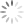Say hello to you!w568w 创建时间被隐藏

2023 年 1 月 27 日更新：

2023 年 2 月 13 日更新：

$\lim_{学习 \to 坚持} 学习=成功$

# 参考书籍

1. 《高等数学（第六版）》上册
同济大学数学系 编
高等教育出版社 出版
2. 《7天搞定微积分》
石山平 大上丈彦 著 李巧丽 译
南海出版公司 出版
3. 《Principles of Mathematical Analysis》
Walter Rudin 著
McGraw-Hill Education 出版

# 我是否适合看下去?

1. 至少初中水平的数学应用能力
2. 至少小学水平的阅读能力
3. 耐心和对数学的热情
4. 以上三条是扯淡
5. 这条也是

# 一、导数

导数是微分学的主要内容。翻回去看看百度百科对导数的解释：

## 1.1 First Blood —— 斜率哈哈，开个玩笑，还是先别急着关掉页面(从哪里能找到这么没节操的文章 真是)。

### 1.1.1 [选读]斜率的严格定义

（对于初三及以上水平的读者，可以有选择性地阅读这些标有「[选读]」文字的部分，因为这些部分可以加深对一些知识的理解。如果你不想看，跳过也没关系

slope，又称「角系数」，是一条直线对于横坐标轴正向夹角的正切，反映直线对水平面的倾斜度。如图，$$直线P_1P_2$$的斜率即为$$k=\tan\alpha=\frac{y\_2-y\_1}{x\_2-x\_1}$$。 其中$$α$$又被称为倾斜角，取值范围为$$0^\circ \leq \alpha<180^\circ$$

## 1.2 点的斜率？

PS: 从这里我开始尝试使用 GeoGebra 来作图，网页版的画图实在是太!蛋!疼!了!。。就这样不断靠近靠近。。终于，在N+N次逼近A点后，三个点几乎实现了重合:注意，我这里之所以强调几乎，是因为它们确实没有碰上，只是它们之间的距离已经微小到了无穷的小。

(假设你已经看明白了，如果没有，你就假装看懂了 :) )

OK，那么接下来，让我们正式开始导数的学…哦不！可能还要加点料才行….

## 1.3 极限？

1000m
∨
500m
∨
200m
∨
100m
∨
1m
∨
0.1m
∨
0.01m
∨
0.0000000000001m
...
∨
0.0000000000000000000000000001m


$\lim_{距离 \to 0 m}$

OKOK，理解了，那么让我们在式子上再加点花样吧!

$\lim_{x \to a} f(x)=b$

$y=2x$

$当x=1时,y=2$

$f(x)=2x$

$f(1)=2$

（顺便说一句，这里不一定必须用$$f$$作为函数的标识，如果你喜欢，也可以写

$我真是酷毙了(x)=2x,我真是酷毙了(1)=2$

### 1.3.1 小练习

#### 第一题

$\lim_{x \to 1} x=？$

#### 第二题

$\lim_{x \to 3} x^2=？$

#### 第三题

$\lim_{x \to 0} (2x^2+5x+8)=？$

#### 第四题

$\lim_{x \to 1} \frac{x^2-3x+2}{x-1}=？$

$\frac{x^2-3x+2}{x-1}=\frac{(x-2)(x-1)}{x-1}=x-2$

$\lim_{x \to 1} (x-2)=？$

### 1.3.2 额外的问答时间

$f(x)=x,\lim\limits_{x \to 1} f(x)=1$

## 1.4 斜率的计算

$AC的斜率=\frac{C点纵坐标-A点纵坐标}{C点横坐标-A点横坐标}$一次函数的斜率是某两点的竖直高度差除以水平高度差。类似地，AC的斜率我们也可以这么写。

$AC的斜率=\frac{C点纵坐标-A点纵坐标}{C点横坐标-A点横坐标}=\frac{f(x+h)-f(x)}{(x+h)-x}=\frac{f(x+h)-f(x)}{h}$

$AC的斜率=\frac{f(1+h)-f(1)}{h}$

$AC的斜率=\frac{f(1+h)-f(1)}{h}=\frac{(1+h)^2-1}{h}$

$AC的斜率=\frac{(1+h)^2-1}{h}=\frac{h^2+2h+1-1}{h}=\frac{h^2+2h}{h}=h+2$

$AC的斜率=\lim_{h \to 0}(h+2)$

$AC的斜率=\lim_{h \to 0}(h+2)=2$

### 1.4.1 小结

1. 在要求斜率的点$$(x,f(x))$$附近找出一个与它很靠近的点$$(x+h,f(x+h))$$
2. 把斜率用$$\frac{C点纵坐标-A点纵坐标}{C点横坐标-A点横坐标}$$表示出来！
3. 在式子前面加上$$\lim_{h \to 0}$$，代入进去计算斜率！

### 1.4.2 通式

$斜率=\lim_{h \to 0}{\frac{f(x+h)-f(x)}{(x+h)-x}}=\lim_{h \to 0}{\frac{f(x+h)-f(x)}{h}}$

### 1.4.3 求斜率有什么用？

$g(x)=2x+b$

$g(1)=2*1+b=1$

$b=-1$

$g(x)=2x-1$

## 1.5 导函数

$\lim_{h \to 0}{\frac{f(x+h)-f(x)}{h}}$

$f^{\prime}(x)=\lim_{h \to 0}{\frac{f(x+h)-f(x)}{h}}=\lim_{h \to 0}{\frac{(x+h)^2-x^2}{h}}$

$=\lim_{h \to 0}{\frac{x^2+2xh+h^2-x^2}{h}}=\lim_{h \to 0}{\frac{2xh+h^2}{h}}$

$=\lim_{h \to 0}(2x+h)$

$=\lim_{h \to 0}(2x+0)=2x$

$A的斜率=f^{\prime}(1)=2$

$B的斜率=f^{\prime}(2)=4$

$$x$$对应的函数值$$y$$ 是函数的值 是函数$$f(x)$$的图像上，横坐标为$$x$$的这一点的求导结果

(注：零点：函数的零点就是$$函数=0$$这个方程的解。 比如$$f(x)=x^2-1$$的零点就是$$x^2-1=0$$的解，也就是$$1$$$$-1$$ 。）

$$f(x)=ax^2+bx+c$$为例。它求导的结果是$$f^{\prime}(x)=2ax+b$$

$$f^{\prime}(x)=0$$的解就是$$x=-\frac{b}{2a}$$。这是不是与你在课堂上学到的二次函数的最值完全一致？

1. 求导，得到$$f^{\prime}(x)=2x$$.
2. 画出$$f^{\prime}(x)=2x$$的图像。3. 准备开始画图！先找出一个起点，这里我们取$$f(0)$$这一点，也就是$$A(0,2)$$4. 按照$$f^{\prime}(x)$$的图像所示，y轴的右边应该都是上升的，而且斜率越来越大，也就是：5. 同理，画出左半边：### 1.5.1 导数的表示方法

$y=a+b$

$$a$$$$b$$，到底谁才是自变量？谁是常量？

$$y=f(x)$$关于$$x$$求导可以表示成：

$\frac{dy}{dx},\frac{d}{dx}y,\frac{df(x)}{dx},\frac{d}{dx}f(x)$

(正因如此，他的符号至今仍在大学数学中频频现身。)

$\frac{d}{dx}x^2=2x$

$f^{\prime\prime}(x)=\frac{d}{dx}\times\frac{d}{dx}\times y=\frac{d^2y}{dx^2}$

### 1.5.2 小结&补充

1. 斜率是什么&怎么算
2. 导数是什么&怎么求
3. 导函数是什么&怎么求

$b^\prime=0$

$x^\prime=1$

$(kx)^\prime=k$

$(kx+b)^\prime=k$

$(ax^n)^\prime=anx^{n-1}$

$y^{\prime}=2*999*x^{999-1}=1998x^{998}$

[选读]这里还有一些为学过三角函数的同学补充的：

$(sinx)^\prime=cosx$

$(cosx)^\prime=-sinx$

$(tanx)^\prime=\frac{1}{(cosx)^2}$

### 1.5.3 五年高考，三年模拟(雾)1. 求出$$f(x)=x^4-2x$$的导函数。
2. 求出$$f(x)=(x+2)^{11}$$的导函数。
3. 求出$$f(x)=x^{x}$$的导函数。
4. 求出$$f(x)=\sqrt{x}$$的导函数。
5. 求出$$f(x)=\sqrt[x]{x}$$的导函数。
6. 求出$$f(x)=\sqrt[sin(x)]{x^{cos(x)}}$$的导函数。
7. 对于以上题目，你有什么感想？

2021年9月24日更新：作者回来啦！现在已经是一条大二狗了，国庆期间会重启更新~

### 1.5.4 导函数的四则运算

$$f(x)=ax^2+bx+c$$为例。它求导的结果是$$f^\prime(x)=2ax+b$$

（限时挑战任务！看看你能不能在 10 秒内找到这句话的出处:) ）

$(A+B)^\prime=A^\prime+B^\prime$

（别忘了一撇「$$\prime$$」的含义哦，它是指对这个式子求导。）

（大家读到这里可能比较突兀，因为上面的练习里面忘了出相关的题了。偏偏这个乘法运算又很重要，还是希望大家快速过一遍！不要跳过哈，不然你待会儿会后悔的，哼哼）

$f(x)=(x^3+3x+3)(x^2+2x+2)$

$f^\prime(x)=(x^3+3x+3)^\prime(x^2+2x+2)+(x^3+3x+3)(x^2+2x+2)^\prime$

$=(3x^2+3)(x^2+2x+2)+(x^3+3x+3)(2x+2)$

$(AB)^\prime=AB^\prime+A^\prime B$

$(f(x)g(x))^\prime=f^\prime(x)g(x)+f(x)g^\prime(x)$

[选读] 加法求导公式的证明

$f^{\prime}(x)=\lim_{h \to 0}{\frac{f(x+h)-f(x)}{h}}$

\begin{aligned}(f(x)+g(x))^\prime\\&=\lim_{h \to 0}{\frac{(f(x+h)+g(x+h))-(f(x)+g(x))}{h}}\\&=\lim_{h \to 0}{\frac{f(x+h)+g(x+h)-f(x)-g(x)}{h}}\\&=\lim_{h \to 0}{\frac{(f(x+h)-f(x))+(g(x+h)-g(x))}{h}}\\&=\lim_{h \to 0}{\frac{f(x+h)-f(x)}{h}}+{\frac{g(x+h)-g(x)}{h}}\\&=f^{\prime}(x)+g^{\prime}(x)\end{aligned}

[选读] 乘法求导公式的证明

$(f(x)g(x))^{\prime}=\lim_{h \to 0}{\frac{f(x+h)g(x+h)-f(x)g(x)}{h}}$

\begin{aligned}(f(x)g(x))^{\prime}\\&=\lim_{h \to 0}{\frac{f(x+h)g(x+h)-f(x)g(x)}{h}}\\&=\lim_{h \to 0}{\frac{f(x+h)g(x+h)-f(x+h)g(x)+f(x+h)g(x)-f(x)g(x)}{h}}\\&=\lim_{h \to 0}{\frac{f(x+h)(g(x+h)-g(x))+(f(x+h)-f(x))g(x)}{h}}\\&=\lim_{h \to 0}{f(x+h)\frac{g(x+h)-g(x)}{h}}+\frac{f(x+h)-f(x)}{h}g(x)\\&=f(x+h)g^{\prime}(x)+f^{\prime}(x)g(x)\end{aligned}

PS：这两个证明乍一看很麻烦，但是自己动手写一遍，你会发现还挺简洁的……

2023年2月13日更新：啊，迟来的更新！

1. 对于加法，我们可以用加法求导公式： \begin{aligned}(f(x)+g(x))^\prime&=f^{\prime}(x)+g^{\prime}(x)\end{aligned}
2. 对于乘法，我们可以用乘法求导公式：
\begin{aligned}(f(x)g(x))^{\prime}&=f^{\prime}(x)g(x)+f(x)g^{\prime}(x)\end{aligned}

$(f(x)-g(x))^\prime=f^{\prime}(x)-g^{\prime}(x)$

$f(x)-g(x)=f(x)+(-1)\times g(x),$

（然后你就会知道 $$\left[(-1)\times g(x)\right]'=-1\times g'(x)=-g'(x)$$ 了。要时常回顾之前学过的东西哦！）

### 1.5.5 复合函数的导函数

$g(x)=x+1,$

$$g(f(x))$$的式子理应是：

$g(f(x))=f(x)+1.$

$g(f(x))=x^2+1.$

[提示]

1. $$f(x)$$：代表一个函数，它的自变量是$$x$$
2. $$f(1)$$：代表令函数$$f(x)$$的自变量$$x$$等于1时的函数值
3. $$f(g(x))$$：代表令函数$$f(x)$$的自变量$$x$$等于另一个函数$$g(x)$$时的函数

$f(1)=1^2+y=1+y.$

1. 你塞进去一个未知量，就一定能得知黑盒子的全貌。比如你塞进去一个变量 $$x$$，就能得知这个函数和其他任何量有什么关系，也清楚明白地知道黑盒子的运作原理了：$$f$$ 其实就是把塞进去的东西平方，然后再加上一个未知量 $$y$$ 嘛！
2. 它计算出的东西一定是一个式子。当然，这个式子里可能有整数，有小数，有加减乘除，甚至有未知量，但它一定是一个式子。除此之外，函数不会给你任何保证。你塞进去一个值，它或许会给你一个值，也或许会给你一个式子。

$\left[g(f(x))\right]'=g'(f(x))f'(x).$

[选读] 复合函数求导公式的证明

PS：说复杂，其实也不复杂，主要是有很多边边角角的情况需要分类讨论，每一种单独证明，因此汇成了一篇长篇大论……

1. 先计算$$f(x)$$的导数，得到$$f'(x)=2x$$
2. 再计算$$g(x)$$的导数，得到$$g'(x)=1$$
3. 然后计算$$g'(f(x))$$，得到$$g'(f(x))=1$$（因为 $$g'(x)$$ 是一个常函数，你把 $$x$$ 换成任何值，它都给你 $$1$$！）；
4. 最后，计算$$g'(f(x))f'(x)$$，得到$$g'(f(x))\times f'(x)=1\times 2x=2x$$
5. 所以，最终的答案是$$\left[g(f(x))\right]'=2x$$

$f(x)=x+2, g(x)=x^{11},$

1. 先计算$$f(x)$$的导数，得到$$f'(x)=1$$
2. 再计算$$g(x)$$的导数，得到$$g'(x)=11x^{10}$$
3. 然后计算$$g'(f(x))$$，得到$$g'(f(x))=11(x+2)^{10}$$
4. 最后，计算$$g'(f(x))f'(x)$$，得到$$g'(f(x))\times f'(x)=11(x+2)^{10}\times 1=11(x+2)^{10}$$
5. 所以，最终的答案是$$\left[g(f(x))\right]'=11(x+2)^{10}$$

### 1.5.6 导函数的除法运算

$\frac{f(x)}{g(x)}=f(x)\times \left[g(x)\right]^{-1}.$

$$\left[g(x)\right]^{\text{xxx}}$$ 我们习惯上简写成 $$g^{\text{xxx}}(x)$$，这里用方括号括起来是方便你理解的。后面我们就不再用方括号，直接写成 $$g^{\text{xxx}}(x)$$ 了。

「对第一个式子求导，乘第二个」是：

$f^{\prime}(x)\times g^{-1}(x),$

「对第二个式子求导，乘第一个」是：

$f(x)\times \left[g^{-1}(x)\right]',$

$(ax^n)'=nax^{n-1}.$

$f'(x)=\left(x^{-1}\right)'=-1\times x^{-2} = -x^{-2}.$

\begin{aligned} \left(\left[g(x)\right]^{-1}\right)'&=[f(g(x))]'\\ &=f'(g(x))g'(x)\\ &=-\frac{1}{g^2(x)}\times g'(x)\\ \end{aligned}

$f(x)\times \left[g^{-1}(x)\right]'=-f(x)\frac{1}{g^2(x)}g'(x).$

$-f(x)\frac{1}{g^2(x)}g'(x)+f^{\prime}(x)\times g^{-1}(x)$

$\left[\frac{f(x)}{g(x)}\right]'=\frac{f^{\prime}(x)g(x)-f(x)g^{\prime}(x)}{g^2(x)}.$

1. 一些通用的求导公式
2. 加减乘除的求导公式
3. 复合函数的求导公式

PS：下一次更新将在本周内，敬请期待~